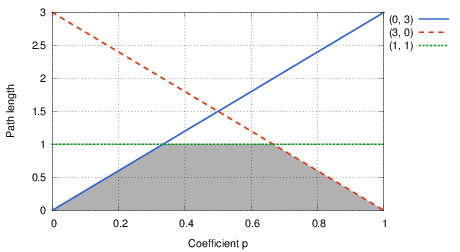# Internet Problem Solving Contest

## Solution to Problem I – Inexpensive Travel

### Easy subproblem

Let us start by examining how the path length depend on the parameter p. For example, consider three parallel roads with (d, a) = (0, 3), (3, 0), and (1, 1), respectively. The plot of the shortest length as a function of p is shown in the following figure:In essence, for each of the roads the length is a linear function of p, hence its plot is a line. The shortest length then corresponds to the lower envelope of these lines – i.e., to the boundary of the intersection of the lower halfplanes determined by our lines. The points pi where a change occurs are the vertices of this polyline.

This would be enough to solve the problem for very small graphs. You could simply enumerate all simple paths from start to finish, for each of them you could compute the total distance and the total ascent, and then you would have as many lines as there were simple paths. The vertices of the lower envelope for those lines would be the answer we seek.

Now we have to make an observation that is similar to how the shortest paths algorithms operate: whenever a path from x to y is “too long”, i.e., if it is not the shortest path for at least one value p ∈ (0, 1), adding an edge y → z will always create a path from x to z that is “too long”.

Therefore, as a general recipe, we can discard “too long” paths as soon as we can determine that we don’t need them. Now, the question is how to construct all the remaining paths. Here, we could again re-use ideas from the standard shortest-path algorithms: These algorithms basically iterate over edges and for each edge x → y they try to shorten the best solution for y using the best solution for x. We can do the same, just instead of a single number (length) we need to remember all combinations of (d, a) that are not “too long”. However, there is a potential caveat here – algorithms such as Dijkstra rely heavily on the particular order in which vertices are processed, and such order is hardly going to be consistent for different distance-vs-ascent tradeoffs. Fortunately, there is a shortest paths algorithm that is oblivious to the ordering – Bellman-Ford. This algorithm just iterates n times over all edges in an arbitrary order. The whole solution is therefore to run Bellman-Ford over all edges, for each edge x → y compute the union of all paths to y with all paths to x extended with the edge x → y, and then throw away all “too long” paths from this set by computing the geometric intersection. The intersection is a standard geometric algorithm that has an efficient implementation using a sweep line.

### Hard subproblem

If we look at the hard subproblem, it looks pretty hard – the limits are really big and there does not seem to be an easy way to improve our previous solution to handle them.

However, it wouldn’t be a real IPSC contest if we did not prepare something special for you. IPSC is an open-data contest – you have the data, examine it. (We even suggested that in the problem statement. Or did you skip that sentence?)

So, what can we learn from the input generator? Let’s look closely at the following code:

``````x = rng.randint(0, n-2)
y = rng.randint(x+1, n-1)
...
print permutation[x], permutation[y], dist, hill``````

Clearly, x and y are generated in such a way that x < y. Therefore, the graph generated by this algorithm is a directed acyclic graph. Computing shortest paths is much easier on such graphs – we just iterate (once) over all vertices in the topological order and we are done.

Oh wait, but now there is this ugly permutation. Does that mean I have to implement topological sort as well? No, you don’t have to. The really cool thing about input generators is that we may actually modify them. In other words, we can print the permutation to the output as well. That way, we do not need to recompute it, just use the already pre-computed value.

Not simple enough? Just get rid of it completely: change the print line to

``print x+1, y+1, dist, hill``

and the permutation is gone. (Note that you still have to generate the permutation because you do need to consume the output of the random generator.)